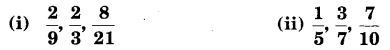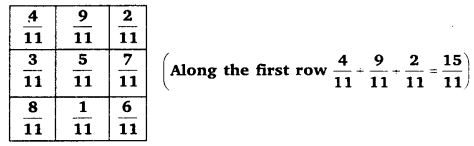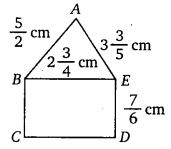# Class 7 Maths NCERT Solutions for Chapter 2 Fractions and Decimals EX – 2.1

## Fractions and Decimals

Question 1.
Solve :Solution:

Question 2.
Arrange the following in descending order :Solution:
We observe that the given fractions neither have a common denominator nor a common numerator. So, first we convert them into like fractions, i.e., fractions having common denominator. For this, we first find the L.C.M. of the denominators of the given fractions.Question 3.
In a “magic square”, the sum of the numbers in each row, in each column and along the diagonals is the same. Is this a magic square?Solution:

⇒ The sum of the numbers in each row, in each column and along the diagonals is the same.
So, the given square is a magic square.

Question 4.
A rectangular sheet of paper is 12$\frac { 1 }{ 2 }$ cm long and 10$\frac { 2 }{ 3 }$ cm wide.Find its perimeter.

Solution:

Question 5.
Find the perimeters of (i) ∆ ABE (ii) the rectangle BCDE in this figure. Whose perimeter is greater?Solution:

Question 6.
Salil wants to put a picture in a frame. The picture is 7$\frac { 3 }{ 5 }$ cm wide. To fit in the frame, the picture cannot be more than 7$\frac { 3 }{ 10 }$ cm wide. How much should the picture be trimmed?

Solution:

Question 7.
Ritu ate$\frac { 3 }{ 5 }$ part of an apple and the remaining apple was eaten by her brother Somu. How much part of the apple did Somu eat? Who had the larger share? By how much?

Solution:

Question 8.
Michael finished colouring a picture in$\frac { 7 }{ 12 }$ hour. Vaibhav finished colouring the same picture in$\frac { 3 }{ 4 }$ hour. Who worked longer? By what fraction was it longer?

Solution: